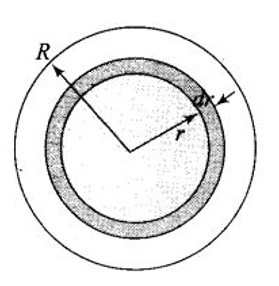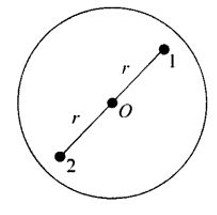# Consider a sphere of radius R with charge density distributed as a) Find the electric field at all points r. (b) Suppose the total charge on the sphere is 2e where e is the electron charge. Where can two protons be embedded such that the force on each of them is zero? Assume that the introduction of the proton does not alter the negative charge distribution.

a) The charge density distribution expression in the sphere tells that the electric field is radial.Let us consider a sphere S of radius R and two hypothetic spheres of radius and .
Let us first consider for point , electric field intensity will be given by,

Here

We get

As charge density is positive, it means the direction of E is radially outwards.

Now consider points , electric field intensity will be given by

Which gives

Here also the charge density is again positive. So, the direction of E is radially outward.

(b) The two proton must be placed symmetrically on the opposite sides of the centre along a diameter. This can be shown by
the figure given below. Charge on the sphere,The proton 1 and 2 are embedded at distance r from the centre of the sphere as shown, then attractive force on proton 1 due to charge
distribution is

and repulsive force on proton 1 due to proton 2 is

Net force on proton 1,

Thus, net force on proton 1 will be zero, when

Hence the protons must be at a distance from the centre.

## Similar Questions

### Preparation Products

##### Knockout NEET May 2021 (One Month)

An exhaustive E-learning program for the complete preparation of NEET..

₹ 14000/- ₹ 6999/-
##### Foundation 2021 Class 10th Maths

Master Maths with "Foundation course for class 10th" -AI Enabled Personalized Coaching -200+ Video lectures -Chapter-wise tests.

₹ 350/- ₹ 112/-
##### Knockout JEE Main April 2021 (One Month)

An exhaustive E-learning program for the complete preparation of JEE Main..

₹ 14000/- ₹ 6999/-
##### Knockout NEET May 2021

An exhaustive E-learning program for the complete preparation of NEET..

₹ 22999/- ₹ 14999/-